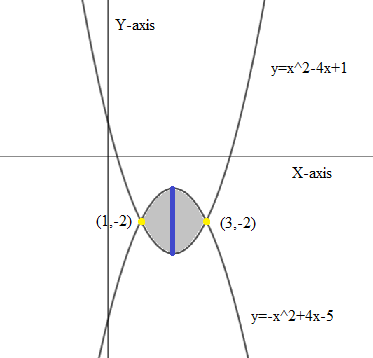# Find the area of the indicated region. Graph the curves to check whether one is above the other...

## Question:

Find the area of the indicated region. Graph the curves to check whether one is above the other or whether they cross, and you use technology to check your answer.

Between {eq}y= x^2 - 4x + 1\ and\ y = -x^2 + 4x - 5 {/eq} for x in [0, 3]

## Area of a bounded Region:

Given a region bounded by two curves {eq}\displaystyle y=f_1(x) {/eq} and {eq}\displaystyle y=f_2(x) {/eq} which are bounded within the x-limits {eq}\displaystyle a\leq x \leq b {/eq}. Then taking a vertical strip we can set up an integral to calculate the area as follows,

$$\displaystyle \text{ Area(A) }=\int_{a}^{b}\int_{f_1(x)}^{f_2(x)} \ dy \ dx\\$$

Please refer the figure given below,Considering a vertical (blue) strip we can see that the variable y is bounded by,

{eq}\displaystyle x^2 - 4x + 1 \leq y \leq -x^2 + 4x - 5 {/eq}

Also the variable x will vary as,

{eq}\displaystyle 1\leq x \leq 3 {/eq}

Note: Here the given x domain is [0,3] and the x-limits of the bounded region are [1,3] which totally lie within the domain so we do not need to make any changes to the x-limits in the integral

Considering the above data we get,

{eq}\displaystyle \begin{align} \text{ Area (A) }&=\int_{a}^{b}\int_{f_1(x)}^{f_2(x)} \ dy \ dx\\ &=\int_{1}^{3}\int_{x^2-4x+1}^{-x^2+4x-5} \ dy \ dx \\ &=\int_{1}^{3}\left[ y\right]_{x^2-4x+1}^{-x^2+4x-5} \ dx\\ &=\int_{1}^{3}\left[ (-x^2+4x-5)-(x^2-4x+1)\right] \ dx\\ &=\int_{1}^{3}[-2x^2+8x-6] \ dx\\ &=\left[ \frac{-2x^3}{3}+\frac{8x^2}{2}-6x\right]_{1}^{3}\\ &=\left[ \left(\frac{-2(3)^3}{3}+\frac{8(3)^2}{2}-6(3) \right)-\left(\frac{-2(1)^3}{3}+\frac{8(1)^2}{2}-6(1) \right)\right]\\ &=\left[ (-18+36-18)-\left( \frac{-2}{3}+4-6\right)\right]\\ &=\left[ 0-\frac{-8}{3}\right]\\ &=\frac{8}{3} \end{align} {/eq}